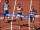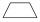Garden

The garden has two opposite parallel fences. Their distance is 33.1 m. Lengths in these two fences are 75.5 meters and 49.4 meters. Calculate the area of this garden.

Result

S =  2067.1 m2

Solution:Leave us a comment of example and its solution (i.e. if it is still somewhat unclear...):Be the first to comment!To solve this example are needed these knowledge from mathematics:

Do you want to convert length units?

Next similar examples:

1. GardenTrapezoid garden has parallel sides 19 m and 24 m. Its area is 193.5 square meters. What is the width of the garden?
2. TrapeziumThe lengths of a parallel sides of a trapezium are (2x+3) and (x+8) and the distance between them is (x+4). if the area of the trapezium is 590 , find the value of x.
3. TrapeziumDetermine the height of the trapezium ABCD, which has area 77.5 cm2 and base lengths 16 cm and 15 cm.
4. Area of trapezoidThe trapezoid bases are and 7 dm and 11 cm. His height is 4 cm. Calculate the area of trapezoid.
5. Mr. TolentinoMr. Tolentino own a piece of land, it measures 86.12 meters long 68.293 meters width. How much longer is the length than the width?
6. SandboxSandbox has area of 32 sq ft and length of 4 1/2 ft. What is width of sandbox.
7. Area of rectangleCalculate the area of rectangle in square meters whose sides have dimensions a = 80dm and b = 160dm.
8. Relay-raceKate ran 170 meters at 29.8 seconds, Suzan at 29.3 seconds, Roza at 34 seconds and Žofka at 30.4 seconds. How long took to run 4x170 m relay-race?
9. Isosceles trapeziumTrapezoid YSED (YS||ED) is isosceles. The size of the angle at vertex Y is 17 degrees. Calculate the size of the angle at vertex E.
10. Whole numbersPavol wrote down a number that is both rational and a whole number. What is one possible number she could have written down?
11. AreaCalculate: ?
12. Area of ditchHow great content area will have a section of trapezoidal ditch with a width of 1.6 meters above and below 0.57 meters? The depth of the ditch is 2.08 meters.
13. Reduce to lowest termsReduce to lowest terms : 32/124
14. Bathroom 2A bathroom is 2.4 meters long and 1.8 meters wide. How many square tiles 1 dm on each side are to be used to cover it?
15. Diameter to areaFind the area of a circle whose diameter is 26cm.
16. Roof tilesThe roof has a trapezoidal shape with bases of 15 m and 10 m, height of roof is 4 meters. How many tiles will need if on 1 m2 should be used 8 tiles?
17. One hectareHow many square meters are one hectare?